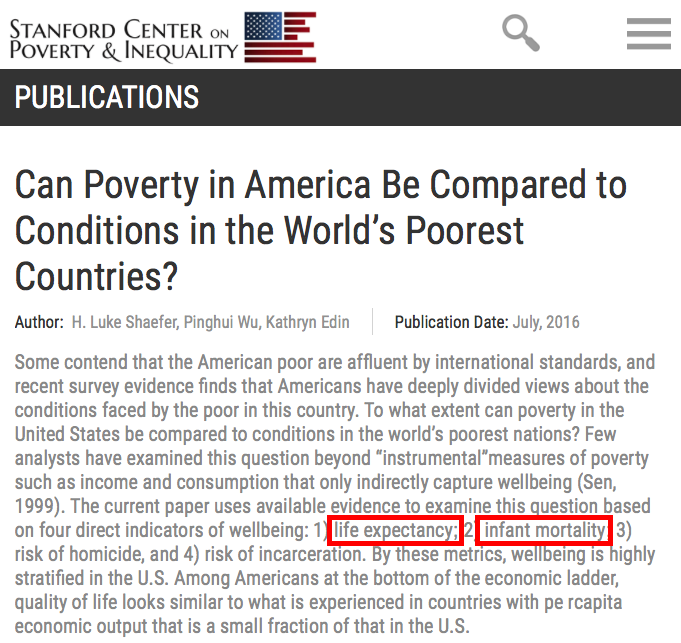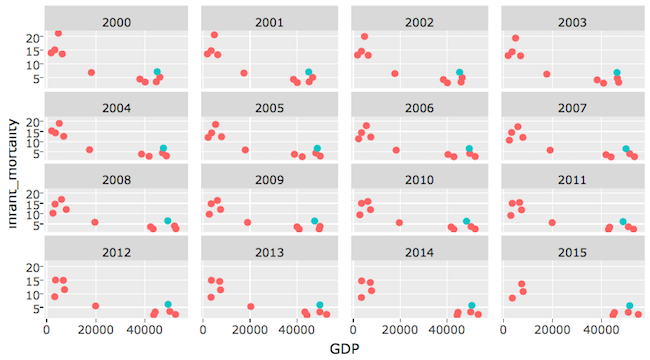## plot

plot(cars)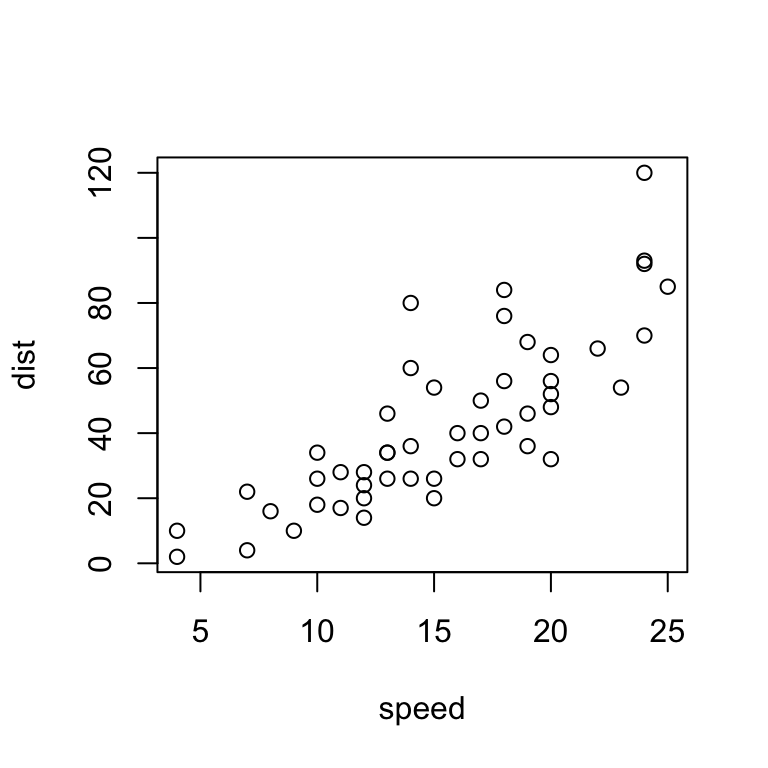## ggplot2

library(ggplot2)
ggplot(cars, aes(speed, dist)) + geom_point()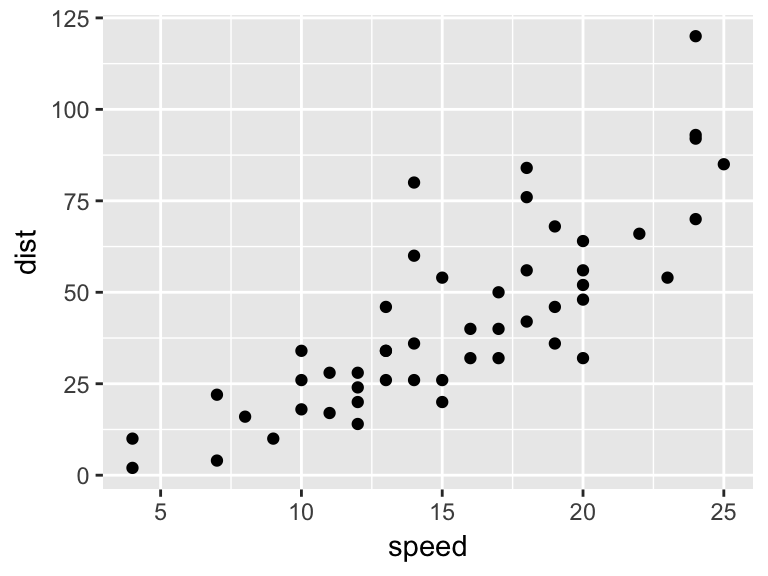The second (2016) edition of the book is here!

## ggthemes

library(ggthemes)
ggplot(cars, aes(speed, dist)) + geom_point() + geom_rangeframe() + theme_tufte()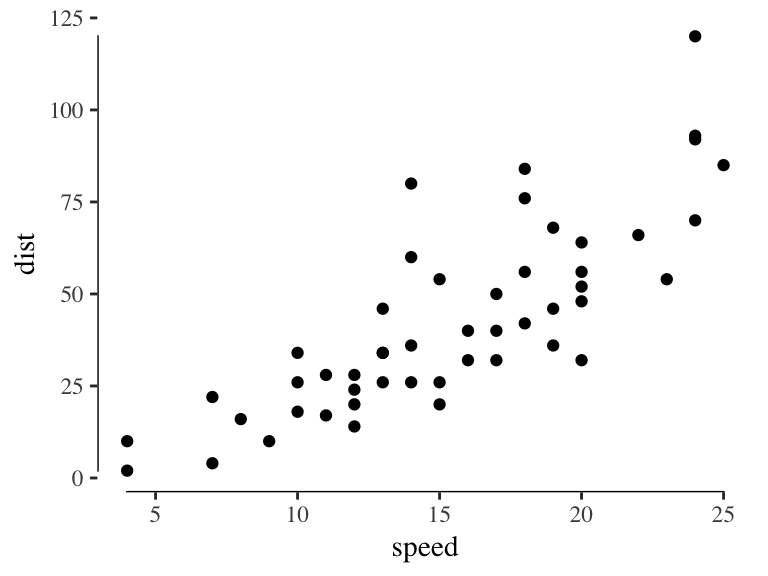## ggiraph

library(ggiraph)
p <- ggplot(cars, aes(speed, dist, tooltip = speed/dist)) + geom_point_interactive()
ggiraph(code = {print(p)}, height_svg = 4)

## ggvis

library(ggvis)
cars %>% ggvis(~speed, ~dist) %>% layer_points()

more on ggvis vs. ggplot

## plotly, using plot_ly function

library(plotly)
plot_ly(cars, x = speed, y = dist, mode = "markers")

## plotly, using ggplotly function

p <- ggplot(cars, aes(speed, dist)) + geom_point()
ggplotly(p)

## shiny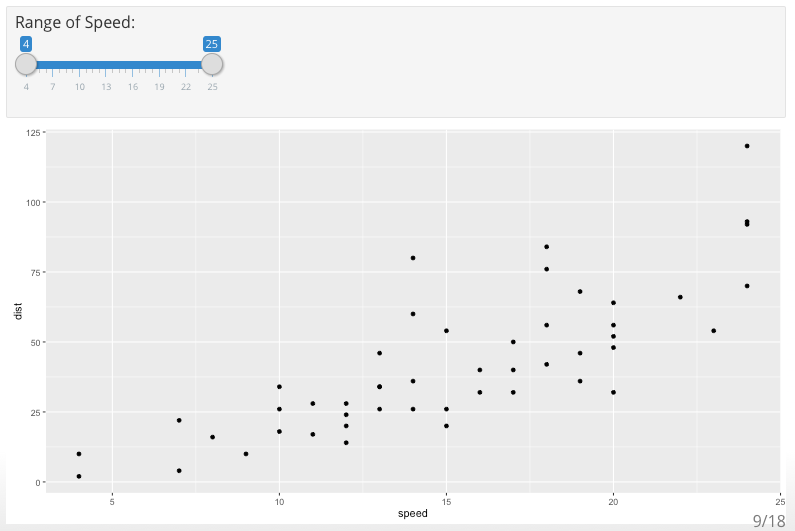## shiny

inputPanel(
sliderInput("speed_range", label = "Range of Speed:",
min = min(cars$speed), max = max(cars$speed),
value = range(cars$speed)) ) renderPlot({ cars_subs <- subset(cars, speed >= input$speed_range &
speed < input\$speed_range)

ggplot(cars_subs, aes(speed, dist)) + geom_point()
})

http://www.showmeshiny.com

## flexdashboard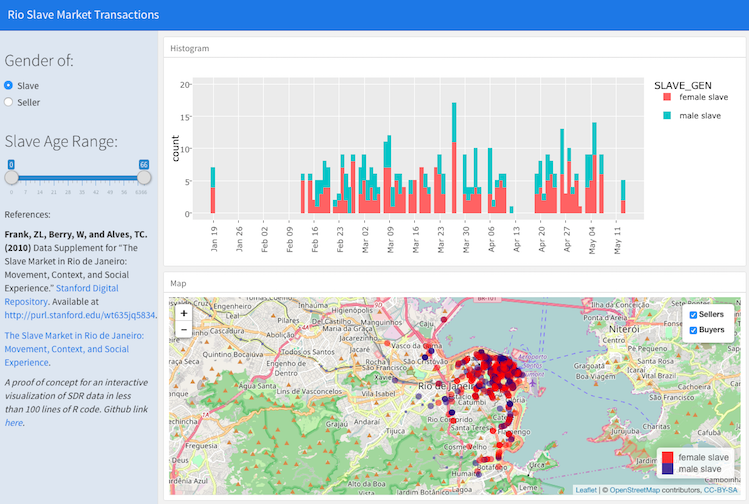https://cengel.shinyapps.io/RioSlaveMarket/

• API (R package)